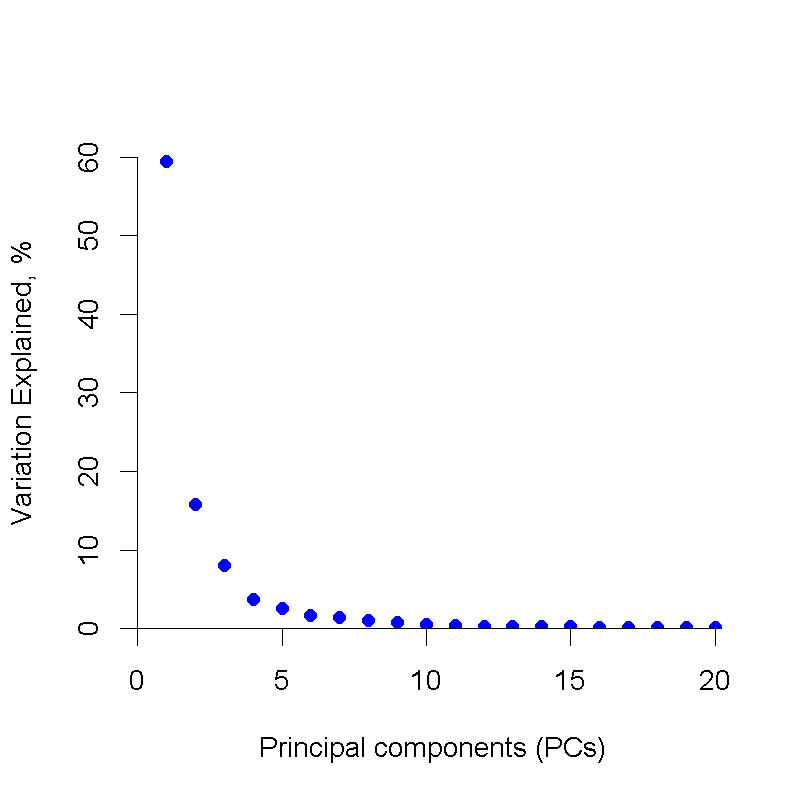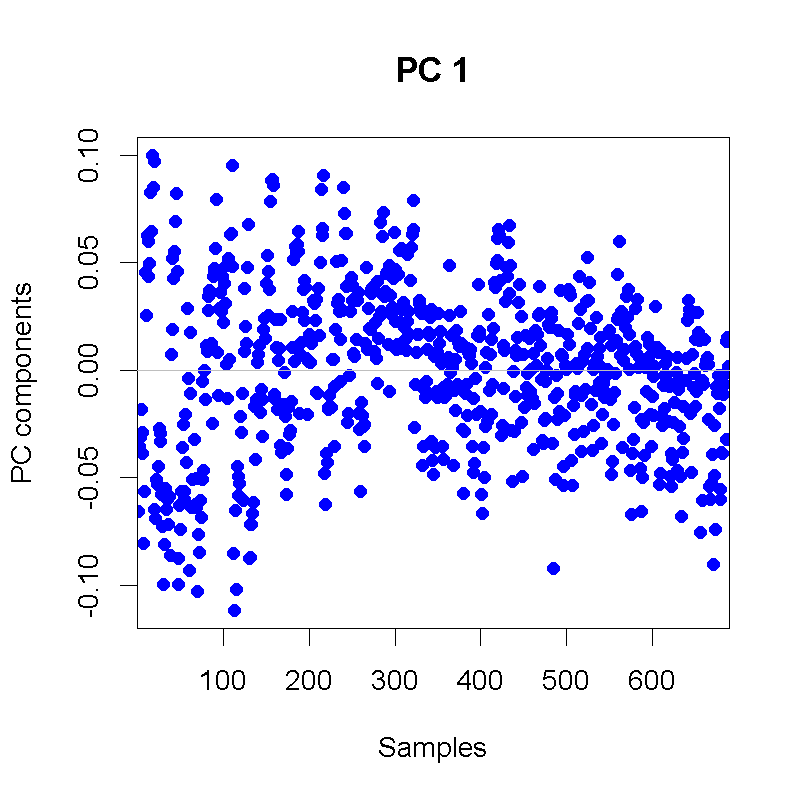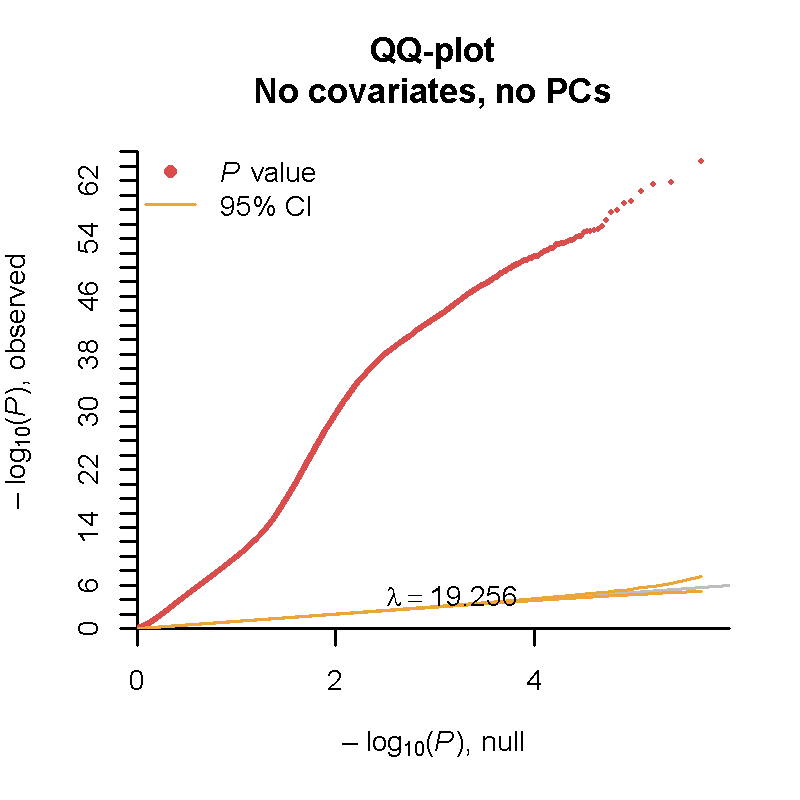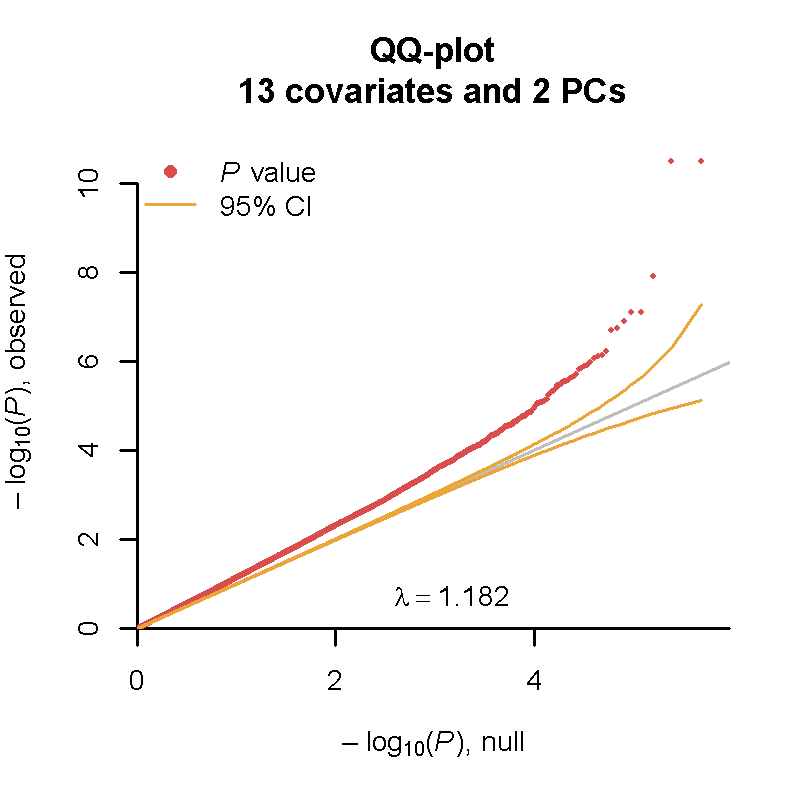# 1 Using RaMWAS with Illumina HumanMethylation450K or MethylationEPIC arrays

RaMWAS can be useful for the analysis of array-based methylation measurements from the Illumina HumanMethylation450K or MethylationEPIC arrays. RaMWAS can perform several quality control steps that are key to prevent test statistic inflation as well as downstream analyses such as principal component analysis (PCA), association testing (MWAS), and multi-marker analysis with cross validation using the elastic net.

## 1.1 Required packages

This vignette makes use of an number of CRAN and Bioconductor packages. The packages can be installed with the following command:

if (!requireNamespace("BiocManager", quietly = TRUE))
install.packages("BiocManager")
BiocManager::install(c(
"minfi",
"IlluminaHumanMethylation450kmanifest",
"IlluminaHumanMethylationEPICmanifest",
"wateRmelon",
"RPMM",
"FlowSorted.Blood.450k",
"FlowSorted.Blood.EPIC"),
update = TRUE, ask = FALSE, quiet = TRUE)

Data from 450k/EPIC arrays can be imported in two ways:

• from raw IDAT files or
• from a file of methylation measures (beta or M-values).

For our example dataset, we will use raw IDAT files from GSE42861.

First we download and untar the data. Be sure the set the working directory to a preferred location with setwd().

download.file(
destfile = 'GSE42861_RAW.tar',
quiet = TRUE,
mode = 'wb')
untar('GSE42861_RAW.tar')

Now we use the minfi package to read the raw IDAT data files into an RGChannelSetExtended object for downstream manipulation.

library(minfi)
destfile = "GSE42861_sampleSheet.csv",
destfilemode = "wb")
targets = read.csv( file = "GSE42861_sampleSheet.csv",
stringsAsFactors = FALSE)
targets = targets,
extended = TRUE,
verbose = TRUE)

To go through the sample code quicker, one can choose to load only a few samples by changing targets = targets to, say, targets = targets[1:20,]. This would limit the example from 689 down to 20.

## 1.4 Probe and sample level quality control

We exclude CpGs and samples based on a number of established criteria.

### 1.4.1 Probes with SNPs and in cross-reactive regions

First, we remove probes in cross-reactive regions and those containing an SNP with minor allele frequency above 0.01 within 10 bp of the single base extension position.

The lists of excluded probes depends on the platform. For Illumina HumanMethylation450k, we use the list provided by Chen et al. (2013). and for Illumina MethylationEPIC (a.k.a. 850k array) we use the list from Pidsley et al. (2016).

The set of excluded probes is stored in exclude.snp.

if( "IlluminaHumanMethylation450k" %in% rgSet@annotation ){
host = "http://www.sickkids.ca/MS-Office-Files/Research/Weksberg%20Lab/"
files = c(
i450k_ns.xlsx = "48639-non-specific-probes-Illumina450k.xlsx",
i450k_pl.xlsx = "48640-polymorphic-CpGs-Illumina450k.xlsx")

for( i in seq_along(files) )
url = paste0(host, files[i]),
destfile = names(files)[i],
mode = "wb",
quiet = TRUE)

ex1 = read_excel("i450k_ns.xlsx", sheet = 1)
ex2 = read_excel("i450k_pl.xlsx", sheet = 1)
ex3 = read_excel("i450k_pl.xlsx", sheet = 2)

exclude.snp = unique(c(
ex1$TargetID, ex2$PROBE,
ex3$PROBE[ (ex3$BASE_FROM_SBE < 10) & (ex3$AF > 0.01)])) rm(host, files, i, ex1, ex2, ex3) } else { host = "https://static-content.springer.com/esm/art%3A10.1186%2Fs13059-016-1066-1/MediaObjects/" files = c( S1_cross_reactive.csv = '13059_2016_1066_MOESM1_ESM.csv', S4_snp_cpg.csv = '13059_2016_1066_MOESM4_ESM.csv', S5_snp_base_extension.csv = '13059_2016_1066_MOESM5_ESM.csv', S6_snp_body.csv = '13059_2016_1066_MOESM6_ESM.csv') for( i in seq_along(files) ) download.file( url = paste0(host, files[i]), destfile = names(files)[i], mode = "wb", quiet = TRUE) snpcpgs1 = read.csv('S1_cross_reactive.csv', stringsAsFactors = FALSE) snpcpgs4 = read.csv('S4_snp_cpg.csv', stringsAsFactors = FALSE) snpcpgs5 = read.csv('S5_snp_base_extension.csv', stringsAsFactors = FALSE) snpcpgs6 = read.csv('S6_snp_body.csv', stringsAsFactors = FALSE) exclude.snp = unique(c( snpcpgs1$X,
snpcpgs4$PROBE, snpcpgs5$PROBE,
snpcpgs6$PROBE[ pmax(snpcpgs6$VARIANT_START - snpcpgs6$MAPINFO, snpcpgs6$MAPINFO - snpcpgs6$VARIANT_END) < 10])) rm(host, files, i, snpcpgs1, snpcpgs4, snpcpgs5, snpcpgs6) } ### 1.4.2 Probes with low bead count We also exclude CpGs with probes having less than 3 beads in more than 1% of the samples (in either red of green channel for Type I probes). The set of probes is stored in exclude.bds. lb = getNBeads(rgSet) < 3 pi1 = getProbeInfo(rgSet, type = "I") pi2 = getProbeInfo(rgSet, type = "II") ex1 = pi1$Name[rowMeans(lb[pi1$AddressA,] | lb[pi1$AddressB,]) > 0.01]
ex2 = pi2$Name[rowMeans(lb[pi2$AddressA,]) > 0.01]
exclude.bds = unique(c(ex1, ex2))
rm(lb, pi1, pi2, ex1, ex2)

### 1.4.3 Probes and samples with low detection p-values

We filter samples and probes using their detection p-values, as calculated by the detectionP function in the minfi package. In this example, samples are dropped if more than 1% of the probes have a detection p-value over 1%. Probes are dropped if more than 1% of the samples have a detection p-value over 1%.

The set of probes is stored in exclude.bds and the set of good samples is stored in keep.samples.

hp = detectionP(rgSet) > 0.01
exclude.hpv = rownames(hp)[rowMeans(hp) > 0.01]
keep.samples = colMeans(hp) < 0.01
rm(hp)

### 1.4.4 Exclusion of low quality samples and probes

rgSet = subsetByLoci(
rgSet = rgSet[,keep.samples],
excludeLoci = c(exclude.snp, exclude.bds, exclude.hpv))

## 1.5 Obtain methylation estimates and save in RaMWAS format

First, we obtain methylation estimates using one of many methods available in the minfi package.

rgSetRaw = fixMethOutliers(preprocessRaw(rgSet))
# beta = BMIQ(rgSetRaw)
beta = getBeta(rgSetRaw)

Next, we save them in a file matrices following RaMWAS standards (see filematrix package). We create data files in the same format as produced by Step 3 of RaMWAS.

The files are saved in rw subdirectory.

dir.create('rw', showWarnings = FALSE)

rng = granges(mapToGenome(rgSet))
chr = seqnames(rng)

# Save CpG locations
library(filematrix)
locs = cbind(chr = as.integer(chr), position = start(rng))
fmloc = fm.create.from.matrix(
filenamebase = paste0("rw/CpG_locations"),
mat = locs,
size = 4)
close(fmloc)
writeLines(con = 'rw/CpG_chromosome_names.txt', text = levels(chr))

# Save estimates
fm = fm.create.from.matrix(
filenamebase = paste0("rw/Coverage"),
mat = t(beta))
close(fm)

## 1.6 Covariates for analysis

To avoid test statistic inflation, we first generate covariates that may capture technical artefacts for possible inclusion in the association tests. This list of covariates is then pruned to only those that affect the methylation data to avoid unnecessary loss of degrees of freedom (i.e., power).

### 1.6.1 Principal components analysis (PCA) on control probes

We extract red and green channel for the control probes.

controlType = unique(getManifest(rgSet)@data$TypeControl$Type)
controlSet = getControlAddress(rgSet, controlType = controlType)
probeData = rbind(getRed(rgSet)[controlSet,], getGreen(rgSet)[controlSet,])

Next we run principal component analysis on the data after light normalization.

data = probeData - rowMeans(probeData)
covmat = crossprod(data)
eig = eigen(covmat)

The number of principal components included as covariates is usually determined by the scree plot.

library(ramwas)
plotPCvalues(eig$values, n = 20) plotPCvectors(eig$vectors[,1], i = 1, col = 'blue')nPCs = 2
covariates.pca = eig$vectors[,seq_len(nPCs)] colnames(covariates.pca) = paste0('PC',seq_len(nPCs)) rm(probeData, data, covmat, eig, nPCs)  ### 1.6.2 Cell type composition For DNA derived from whole blood, cord blood, or brain tissue, we can estimate cellular proportions using the estimateCellCounts() function in the minfi package. In this example, we estimate cell proportions in blood. covariates.cel = estimateCellCounts( rgSet = rgSet, compositeCellType = "Blood", cellTypes = c("CD8T", "CD4T", "NK", "Bcell", "Mono", "Gran"), meanPlot = FALSE) ### 1.6.3 Median methylated and unmethylated intensity covariates.umm = getQC(rgSetRaw) ### 1.6.4 Phenotypic covariates from the sample sheet We also add age, sex, case-control status, and other covariates from the samples sheet to the covariate data frame. rownames(targets) = targets$Basename;
targets$xSlide = paste0('x',targets$Slide) # Force Slide to be categorical
covariates.phe = targets[
rownames(covariates.umm),
c("xSlide", "Array", "caseStatus", "age", "sex", "smokingStatus")]

# 2 Running RaMWAS on the data

## 2.1 Set up parameters and covariates

To run PCA with RaMWAS we specify three parameters:

• dircoveragenorm – directory with the data matrix
• covariates – data frame with covariates
• modelcovariates – names of covariates to regress out
covariates = data.frame(
samples = rownames(covariates.umm),
covariates.umm,
covariates.pca,
covariates.cel,
covariates.phe)

library(ramwas)
param = ramwasParameters(
dircoveragenorm = 'rw',
covariates = covariates,
modelcovariates = NULL,
modeloutcome = "caseStatus",
modelPCs = 0)

## 2.2 Covariate pruning

To avoid unnecessary loss of degrees of freedom (i.e., power) one should iteratively prune covariates prior to association testing if they are not correlated with the top PCs of the methylation data. During these iterations, the methylation data is residualized using the included covariates prior to performing PCA so that the resulting PCs only capture possible remaining sources of variation.

## 2.3 MWAS without covariates (high inflation factor)

Performing MWAS without correction for any covariates causes high inflation of the test statistics (19.256 here, 15.17 in the original study).

param$modelcovariates = NULL param$modelPCs = 0
ramwas4PCA(param)
ramwas5MWAS(param)
qqPlotFast(getMWAS(param)$p-value) title('QQ-plot\nNo covariates, no PCs')QQ-plot, no covariates, no PCs ## 2.4 MWAS with all covariates (moderate inflation factor) Inclusion of all covariates reduces the inflation of the test statistics from 19.256 down to 1.182. param$modelcovariates = c(
"age", "sex", "Array", "xSlide",
"mMed", "uMed",
"PC1", "PC2",
"CD8T", "CD4T", "NK", "Bcell", "Mono", "Gran")
param$modelPCs = 2 ramwas4PCA(param) ramwas5MWAS(param) qqPlotFast(getMWAS(param)$p-value)
title('QQ-plot\n13 covariates and 2 PC')QQ-plot, 13 covariates and 2 PCs

## 2.5 Further steps of RaMWAS pipeline

Steps 6 and 7 of the RaMWAS pipeline can also be applied to the data matrix exactly as described in the overview vignette.